# Question regarding the output for AC circuit with capacitors

## Homework Statement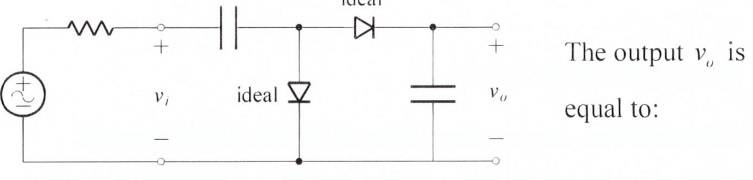Both diodes are ideal.

(a) Vi
(b) -Vi
(c) -2Vi
(d) none of the above

Q=CV

## The Attempt at a Solution

1. For t=0 to Pi:
Cap1 and Cap2 are in series and hence Q1=Q2, therefore Vc1 = Vc2.

From this we get Vi = Vc1 + Vc2, hence Vc1 =Vc2 = Vi/2.

2. For t=Pi to 2Pi,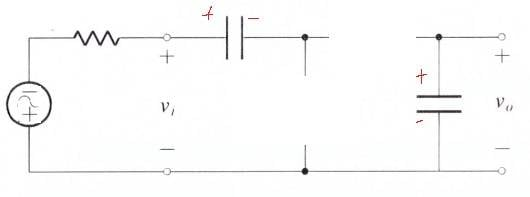The circuit should look like 3 batteries in series as such: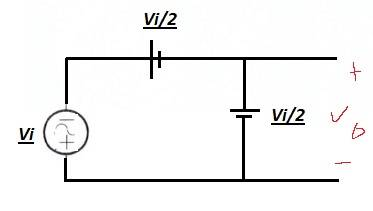,

Therefore Vo = -(Vi + Vi/2 + Vi/2) = -2Vi,
And so the answer is (c).

BUT...
Couldn't the circuit look like this too?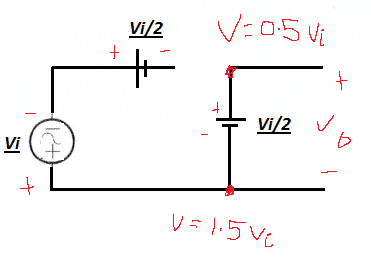And so Vo = - (1.5Vi - 0.5Vi) = -Vi
Thus the answer is (b) ?

BUT...
We are just taking the voltage drop across the resistor, so Vo= Vc2 = 1/2Vi

Which is correct and what exactly am I misunderstanding?

Last edited:

CWatters
Homework Helper
Gold Member
1. For t=0 to Pi:
Cap1 and Cap2 are in series and hence Q1=Q2, therefore Vc1 = Vc2.

Check that. What about the left hand diode?

(I assume you mean Vi is +ve during t=0 to Pi)

CWatters
Homework Helper
Gold Member
It's worth spending a moment just looking at the two diodes. What do the diodes mean for the polarity of the output voltage? Think.. What would need to happen to make it +ve? What would need to happen to make it go -ve.

I hope this is a step in the right direction,

1. For the first positive cycle, Vc1 = Vi and Vc2 does not charge is there is a short circuit due to the left ideal diode
2. For the first negative cycle, Vc2 = Vi + Vc1 = 2Vi. As the polarity of the output terminals reversed, the output should be -(2Vi).
3. For any positive cycle afterwards, the Vo remains -(2Vi) due to the short circuit from left diode.
4. For any negative cycle afterwards, Vo remains -(2Vi) as verified from 2.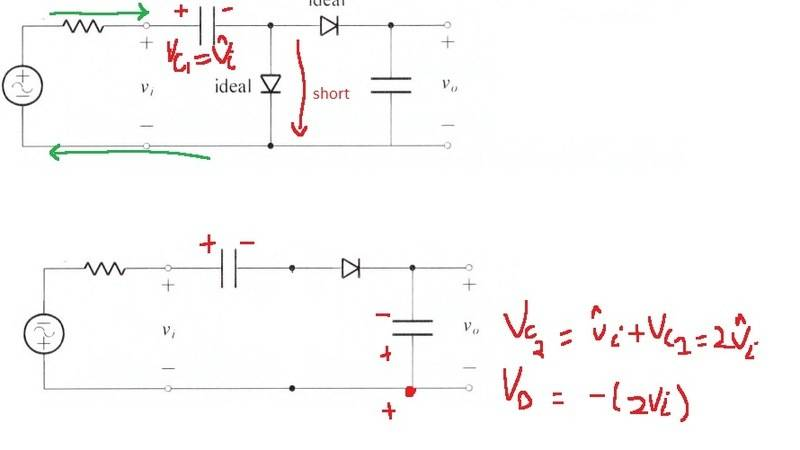CWatters
Homework Helper
Gold Member
2. For the first negative cycle, Vc2 = Vi + Vc1 = 2Vi. As the polarity of the output terminals reversed, the output should be -(2Vi).

Think about the right hand diode. How does a -ve cycle affect the output at all?

Think about the right hand diode. How does a -ve cycle affect the output at all?

The diode is reverse biased, so no current flow. Hence Vc2 to remains uncharged also and Vo is 0 for both the positive and negative cycle?

•CWatters
CWatters
Homework Helper
Gold Member
That's what I think. To get Vo = -Vi (or -2Vi) the output diode has to be the other way around like this..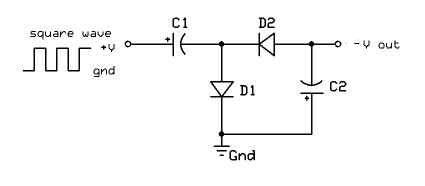•karan000
That's what I think. To get Vo = -Vi (or -2Vi) the output diode has to be the other way around like this..